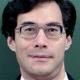0

# Shinichi Mochizuki of Japan Cracks The ABC Conjecture

 Earn up to ₹40 per post as scholarship. Unlimited earning potential per month.Exchange career information, knowledge, interview questions, campus tests, notes & tutorials, give/get answers...A Japanese mathematician, Shinichi Mochizuki, a scholar at Kyoto University, has claimed to have cracked “the abc conjecture”, one of the world’s most complex mathematical theories. Shinichi has released four papers on the internet describing his proof of “the abc conjecture”. He took four years to calculate the theory and if confirmed it would be one of the greatest mathematical achievements of this century. It will take a further time in many more years to confirm the breakthrough as Mochizuki has created an entirely new mathematical language to explain the steps and the others would have learn it to understand the process.

The “abc conjecture” was first proposed by British mathematician David Masser, working with France’s Joseph Oesterle, in 1985, but was never proven.

The “abc conjecture” refers to equations of the form a + b = c. It involves concept of a square-free number, meaning a number that cannot be divided by the square of any number. For instance, 15 and 17 are square-free numbers, but 16 is not as it is divisible by 4 which is a square of 2.

From that, the “abc conjecture” concerns a property of the product of the three integers abc. The conjecture states that for integers a + b = c, the square-free part always has a minimum value greater than zeor and nearly always greater than 1.

Mochizuki however, does not describe himself as a mathematician but as a “inter-universal geometer” and – as long as his theory stands up to the scrutiny – there are hopes that his findings will settle a number of complex problems in number theory and other branches of maths.

Filed in: Latest Education News Tags: ,